### Micro Genetic Algorithm (μGA)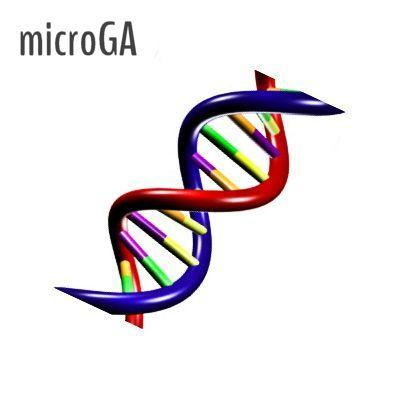## Introduction

xlOptimizer implements μGA (microGA), a variant of Genetic Algorithms that utilizes a very small population (hence the name). It was proposed by Goldberg  and first implemented by Krinsnakumar .

## How does it work?

The algorithm utilizes a very small population of candidate solutions (typically 5-10 individuals) and no mutation. The evolution is performed with crossover only. At some point, the population tends to become homogeneous, featuring individuals with small differences in their genetic material. To overcome this state of stagnation, a restart of the population is performed; only the best individual is kept and the rest are replaced by randomly generated individuals. Typically, a restart of the population is enforced when the ratio of different bits becomes less than 5%.

## Mathematical formulation

The mathematical formulation is similar to the one described for Genetic Algorithms

## Options in xlOptimizer

The following options are available in xlOptimizer:

#### Step 1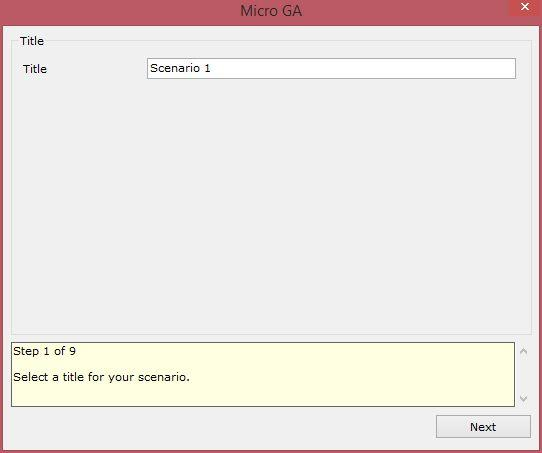Enter a short title for the scenario. The log files will be saved to a subfolder with this name.

#### Step 2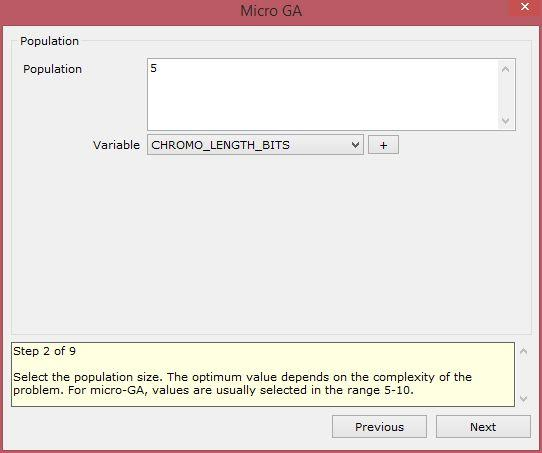Enter the population size for the GA. For Micro GA, usually 5-10 individuals is adequate.

• Example 1: entering '50' sets the population size to 50 individuals.
• Example 2: entering 'IF(CHROMO_LENGTH_BITS / 2 < 100, CHROMO_LENGTH_BITS / 2, 100)' sets the population size to the chromosome length divided by two if the result is less than 100, or 100 otherwise.

The variables that may be used in the expression are:

• CHROMO_LENGTH_BITS: the chromosome length in bits.
• CHROMO_LENGTH_GENES: the chromosome length in genes. This equals the number of design variables.

#### Step 3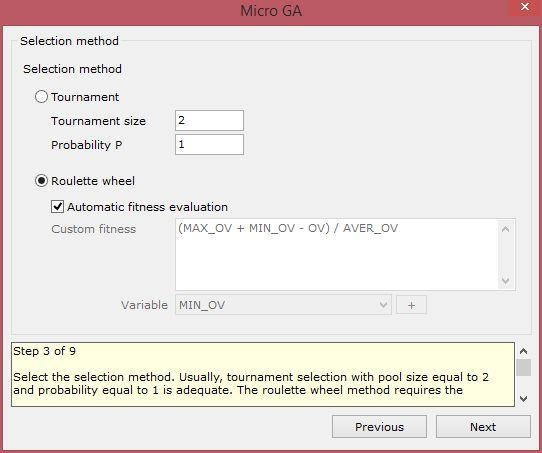Choose the selection method. The method can be either tournament selection or biased roulette wheel. Usually, tournament selection with pool size equal to 2 and probability equal to 1 is adequate. The roulette wheel method requires the definition of a fitness function, based on the objective function. The evaluation of the fitness can be performed automatically, or based on custom code. In the latter case, the variables that can be used are the following:

• MIN_OV: the minimum objective value within the members of the population.
• MAX_OV: the maximum objective value within the members of the population.
• AVER_OV: the average objective value within the members of the population.
• BEST_OV: the best objective value within the members of the population.
• WORST_OV: the worst objective value within the members of the population.
• OV: the objective value of the current individual for which the fitness is being evaluated.
• TARGET: the target objective value for target - problems.

The automatic evaluation is based on the following code:

• '(OV - MIN_OV) / AVER_OV' for maximization problems,
• '(MAX_OV + MIN_OV - OV) / AVER_OV' for minimization problems,
• '(ABS(MAX_OV - TARGET) + ABS(MIN_OV - TARGET) - ABS(OV - TARGET) / ABS(AVER_OV - TARGET)' for target-value problems.

Based on the above, a first level of normalization is achieved with the division by the average objective value.

#### Step 4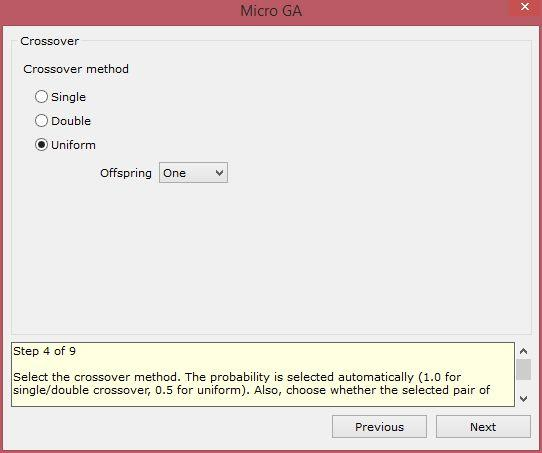Select the crossover method. Usually single crossover with probability 0.7 or uniform crossover with probability 0.5 is selected. Also, choose whether the selected pair of chromosomes will produce one or two offspring.

#### Step 5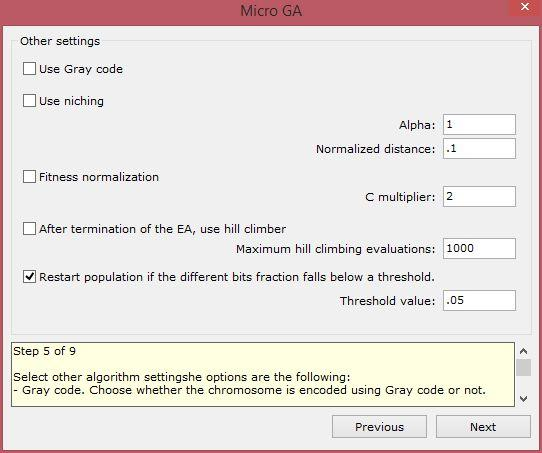Select other algorithm settings. The options are the following:

• Gray code: Choose whether the chromosome is encoded using Gray code or not.
• Niching: Select the alpha coefficient (power law exponent for sharing function; typically = 1.0) and the normalized distance ( 1/normalized distance can be viewed as the number of regions over which the sharing function should focus). This affects the design variables that have been set to participate in a niching strategy.
• Hill climber: Choose whether after the termination of the GA a local optimizer, namely steepest ascend hill climber, is invoked. Also select the maximum number of function evaluations for the local optimizer.
• Restart population after different bits fraction falls below a threshold: Choose the threshold of different bits fraction (with respect to the current best individual) under which the population must be restarted. This is imperative in micro-GA. Usual value is 0.05, i.e. 5%.

#### Step 6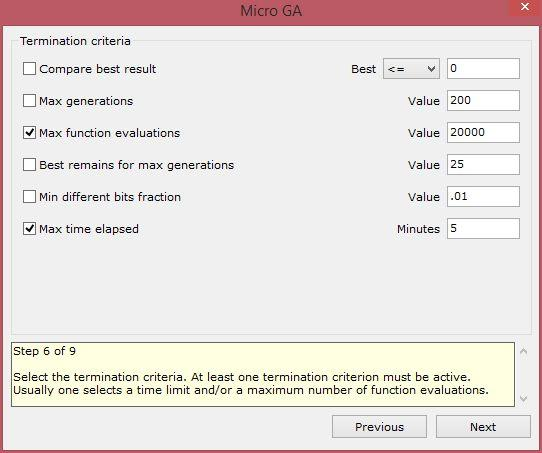Select the termination criteria. At least one termination criterion must be active. Usually one selects a maximum number of function evaluations and/or a time limit.

#### Step 7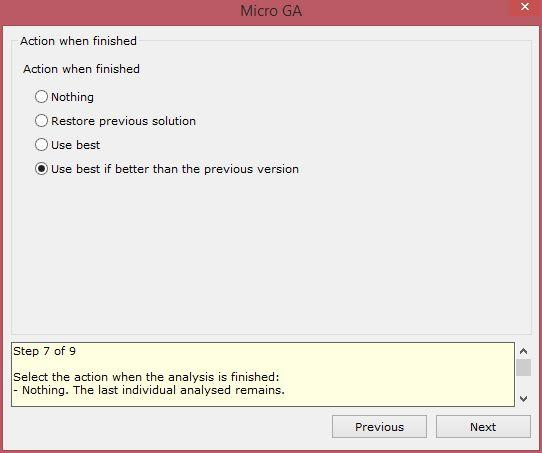Select the action when the analysis is finished:

• Nothing: The last individual analysed remains.
• Restore previous solution: The solution that was visible before the analysis is restored.
• Use best: The best solution that was discovered during the analysis is applied.
• Use best if better than the previous version (recommended): The best solution that was discovered during the analysis is applied only if it is better than the previous solution. In the opposite case the previous solution is restored.

#### Step 8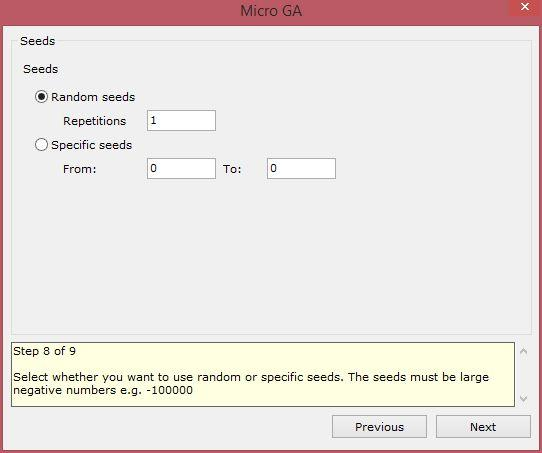Select whether you want to use random or specific seeds for the random number generator. The seeds must be large negative numbers e.g. -100000. Usually a number of analyses with random seeds is performed. The filename of the log is the seed used in the specific analysis.

#### Step 9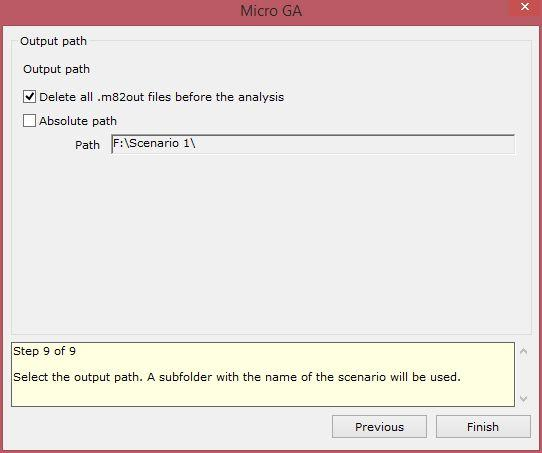Select the output path. A subfolder with the name of the scenario will be used. Usually a relative path is used i.e. the absolute path check box is not checked. This results in the output logs being saved in the same path as the project file.